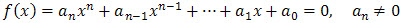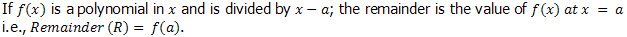# Remainder Theorem

## Introduction

Recall that the value of x  which satisfies the polynomial equation of degree n in the variable x  in the formwhere n  is a positive integer and a0, a1,..., an, are constants, is called a root or solution or a zero of the polynomial. This happens when the remainder is 0 which means that the divisor is a factor of the dividend. However, there are times that the divisor does not divide the dividend exactly and there is a remaining polynomial less than the degree of the divisor which we call the remainder.

To find the remainder when an expression is divided by a given linear expression we use the Remainder Theorem. It states that:Go to the next page to start practicing what you have learnt.